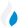•Trade Pi
•Trade
•Exchange
•Trade Pi
•Trade
•Exchange
\$1.12T
Total marketcap
\$79.77B
Total volume
39.44%
BTC dominance
•Trade Pi
•Trade
•Exchange

# dcr to INR Exchange Rate - 1 Decred in INR

2,158.0320532977
• BTC 0.001
• ETH 0.01
Vol [24h]
\$456,558.98

## dcr to INR converter

Exchange Pair Price 24h volume
BitBNS DCR/INR \$26.11 \$455.64K
WazirX DCR/INR \$25.16 \$382.82

## DCR/INR Exchange Rate Overview

Name Ticker Price % 24h 24h high 24h low 24h volume
Decred dcr \$23.68 -4.2608% \$24.88 \$23.68 \$1.88M

Selling 1 Decred dcr you get 2,158.0320532977 INR.

Decred Apr 17, 2021 had the highest price, at that time trading at its all-time high of \$247.35.

663 days have passed since then, and now the price is 9.60% of the maximum.

Based on the table data, the DCR vs INR exchange volume is \$456,558.98.

Using the calculator/converter on this page, you can make the necessary calculations with a pair of Decred to INR.

## Q&A

### What is the current DCR to INR exchange rate?

Right now, the DCR/INR exchange rate is 2,158.0320532977.

### What has been the Decred to INR trading volume in the last 24 hours?

Relying on the table data, the Decred to INR exchange volume is \$456,558.98.

### How can I calculate the amount of INR? / How do I convert my Decred to INR?

You can calculate/convert INR from DCR to INR converter. Also, you can select other currencies from the drop-down list.

## DCR to INR Сonversion Table

DCR INR
0.02 DCR = 43.1 INR
0.04 DCR = 86.3 INR
0.08 DCR = 172.6 INR
0.1 DCR = 215.8 INR
0.2 DCR = 431.6 INR
0.4 DCR = 863.2 INR
0.6 DCR = 1,294.8 INR
2 DCR = 4,316.06 INR
4 DCR = 8,632.1 INR
50 DCR = 107,901.6 INR
500 DCR = 1,079,016.02 INR
5000 DCR = 10,790,160.2 INR
50000 DCR = 107,901,602.6 INR